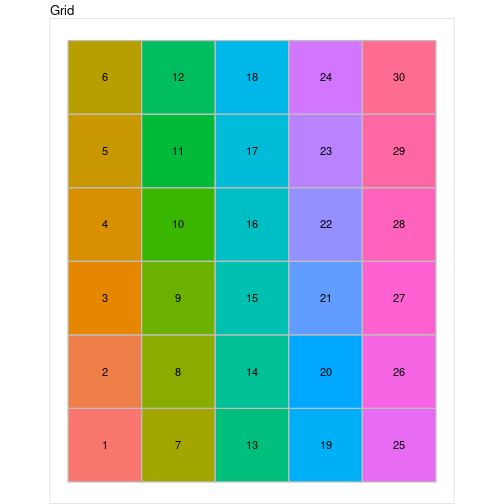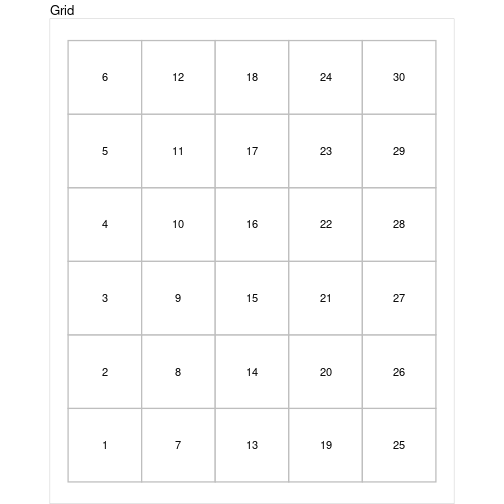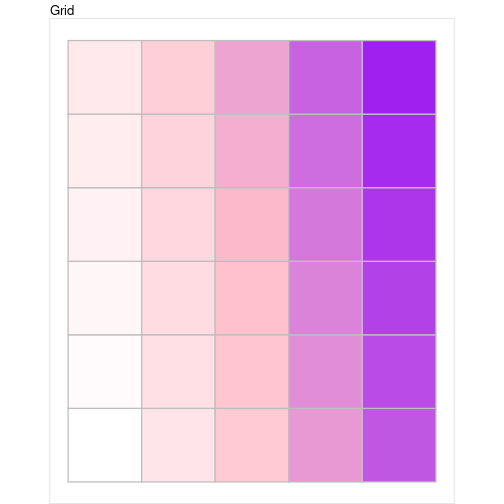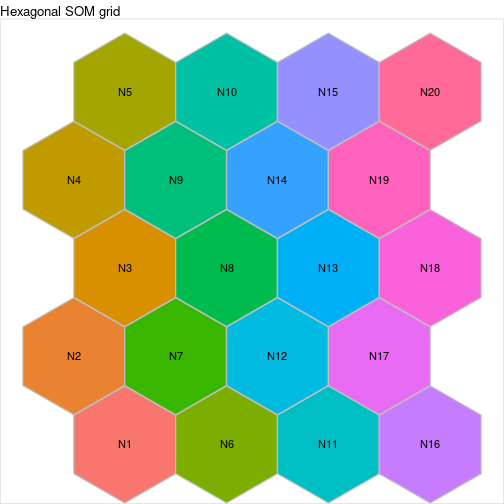## Definition and purpose of a `myGrid` class object

Objects of class `myGrid` are made to display the SOM grid.

## Basic functions on a `myGrid` class object

In this section, we will consider only the four basic functions that can be applied on a `myGrid` class object:

• `initGrid,`
• `print.myGrid`
• `summary.myGrid`
• `plot.myGrid`

### The `initGrid` function

The `initGrid` function initializes a new `myGrid` object. It has 3 arguments:

• `dimension`, which is a vector of two integers. The first one is the x dimension (number of neurons/units on the x axis) and the second one is the y dimension. The default dimensions are x=5 and y=5,

• `topo`, which is the chosen topology. The value of this argument must be one of `square` or `hexagonal`. Thedefault value is square.

• `dist.type`, which is the distance type to compute the distance between two neurons of the grid. The default value is euclidean and the coordinates of the neurons on the grid are chosen so as the minimum Euclidean distance between two neurons is exactly 1. `hexagonal` topoology is only compatible with the Euclidean distance.

The following R code initializes a new `myGrid` object of square topology, x dimension 5 and y dimension 6, and distance type `maximum`.

``````first_grid <- initGrid(dimension = c(5,6), topo = "square", dist.type = "maximum")
``````

### The `print.myGrid` function

The `myGrid` object `print` function prints the main features of the chosen object in the console. The only argument is the object to be printed.

Considering the previously initialized grid, the print command is:

``````print(first_grid)
``````
``````##
##       Self-Organizing Map structure
##
##         Features   :
##            topology     :  square
##            x dimension  :  5
##            y dimension  :  6
##            distance type:  maximum
``````

### The `summary.myGrid` function

The `myGrid` object `summary` function is quite simple. It only prints the class of the object and then calls the print function previously described. The only argument is the object to be summarized.

``````summary(first_grid)
``````
``````##
## Summary
##
##       Class            :  myGrid
##
##       Self-Organizing Map structure
##
##         Features   :
##            topology     :  square
##            x dimension  :  5
##            y dimension  :  6
##            distance type:  maximum
``````

### The `plot.myGrid` function

The `myGrid` object `plot` function draws the squared area corresponding to the object, in a new graphical window. It has 3 parameters:

• the object to be plotted,

• `show.names`, boolean, indicating if the names of the neurons should be displayed on the graph (default to `TRUE`)

• `names`, a vector, giving the names of the neurons if `show.names = TRUE`, default to the number of the neuron.

``````plot(first_grid)
````````````plot(first_grid) + ggplot2::scale_fill_manual(values = rep("white", 30))
````````````my_palette <- colorRampPalette(c("white", "pink", "purple"))(30)
plot(first_grid, show.names = FALSE) +
ggplot2::scale_fill_manual(values = my_palette)
``````Hexagonal grids can be displayed similarly:

``````second_grid <- initGrid(dimension = c(4, 5), topo = "hexagonal")
plot(second_grid, names = paste0("N", 1:20)) +
ggplot2::ggtitle("Hexagonal SOM grid")
``````### Session information

This vignette has been computed with the following environment:

``````sessionInfo()
``````
``````## R version 4.0.2 (2020-06-22)
## Platform: x86_64-pc-linux-gnu (64-bit)
## Running under: Ubuntu 20.04.1 LTS
##
## Matrix products: default
## BLAS:   /usr/lib/x86_64-linux-gnu/blas/libblas.so.3.9.0
## LAPACK: /usr/lib/x86_64-linux-gnu/lapack/liblapack.so.3.9.0
##
## locale:
##   LC_CTYPE=en_US.UTF-8       LC_NUMERIC=C
##   LC_TIME=fr_FR.UTF-8        LC_COLLATE=C
##   LC_MONETARY=fr_FR.UTF-8    LC_MESSAGES=en_US.UTF-8
##   LC_PAPER=fr_FR.UTF-8       LC_NAME=C
##  LC_MEASUREMENT=fr_FR.UTF-8 LC_IDENTIFICATION=C
##
## attached base packages:
##  stats     graphics  grDevices utils     datasets  methods   base
##
## other attached packages:
##  SOMbrero_1.3-1 markdown_1.1   igraph_1.2.5
##
## loaded via a namespace (and not attached):
##   Rcpp_1.0.5           highr_0.8            pillar_1.4.6
##   compiler_4.0.2       tools_4.0.2          digest_0.6.25
##   lattice_0.20-41      lubridate_1.7.9      evaluate_0.14
##  lifecycle_0.2.0      tibble_3.0.3         gtable_0.3.0
##  checkmate_2.0.0      pkgconfig_2.0.3      png_0.1-7
##  rlang_0.4.7          hexbin_1.28.1        xfun_0.16
##  interp_1.0-33        metR_0.7.0           stringr_1.4.0
##  knitr_1.29           generics_0.0.2       vctrs_0.3.2
##  grid_4.0.2           scatterplot3d_0.3-41 glue_1.4.1
##  data.table_1.13.0    R6_2.4.1             farver_2.0.3
##  ggplot2_3.3.2        deldir_0.1-28        magrittr_1.5
##  scales_1.1.1         backports_1.1.8      ellipsis_0.3.1
##  ggwordcloud_0.5.0    mime_0.9             colorspace_1.4-1
##  labeling_0.3         stringi_1.4.6        munsell_0.5.0
##  crayon_1.3.4
``````